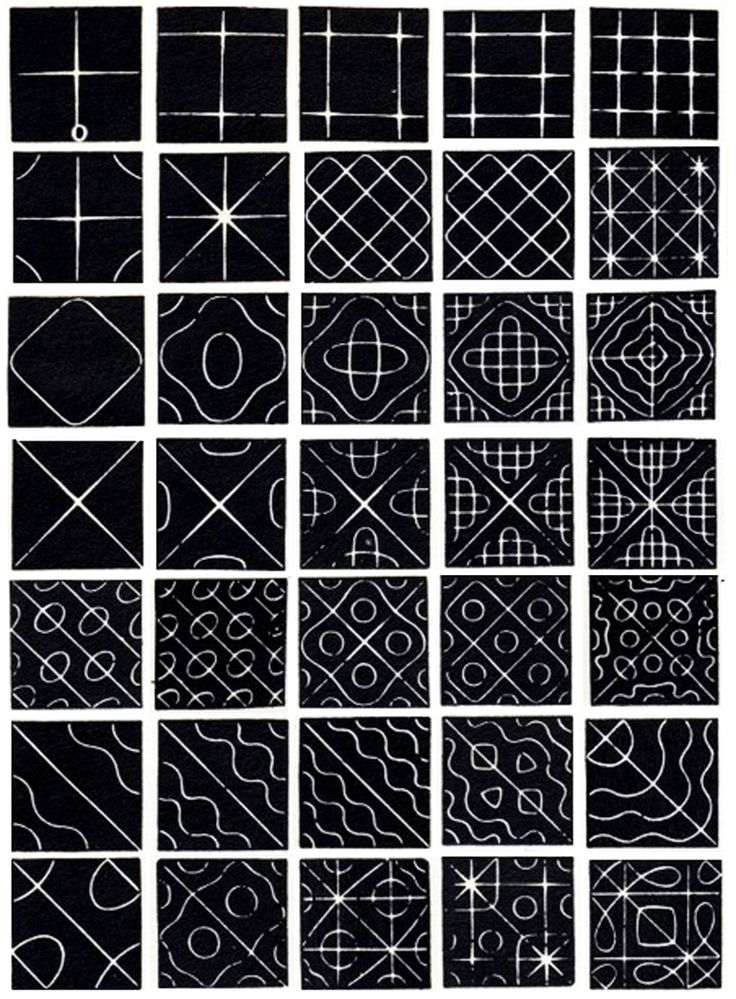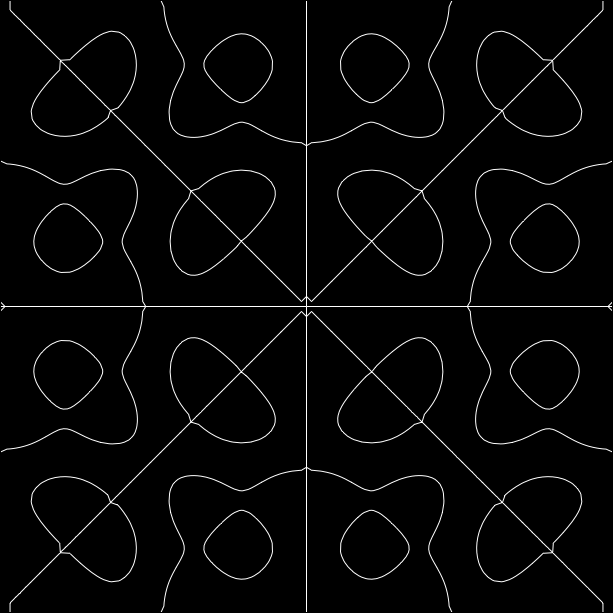# the light

The other day I stumbled across an article by a researcher named Vic Tandy. In the article he recounts a sequence of events that led him to conclude that acoustic standing waves were causing the people in his lab to experience feelings of anxiety and paranormal activity, i.e. visions of apparitions, or ghosts.

This was an amazing story and inspired me to learn more about standing waves, mostly with the intention of pranking friends and family by simulating ghosts. I hopped onto YouTube but soon stumbled upon the following video about Chladni patterns:

At that point I completely forgot about ghost pranks and wondered if instead I could create these Chladni Patterns on my computer without physical equipment.To briefly summarize, Chladni patterns are produced by standing waves over the vibrating plate's surface. The motion of the anti-nodes causes the sand to settle onto the nodes (the stationary points) over the plate, thus creating the Chladni patterns.

### Implementation Strategy

My first idea was to write an active physics simulation and set up the input forces and boundary conditions to match the physical setup that created the Chladni patterns in the video. Numerical simulation of wave propagation over 2D surfaces is pretty well-studied and the simplest implementation can be done in real-time using a finite-difference method over the spatial dimension and a Runge-Kutta method for time propagation. Here's a particularly easy to understand and accesible example implementation.

To draw Chladni patterns with this method, you have to run the simulation in real-time but instead of drawing the entire surface in 3D, you only draw the areas where the height of the wave is zero (the nodes) in 2D. In steady-state this should produce something resembling Chladni patterns.

While I could have just gone ahead and did this, something seemed naggingly wrong with this approach. First, I only really wanted to draw the system when it's in steady-state, so running a real-time simulation seemed like overkill. Second, numerical simulation is fraught with error parameters (discretization error, round-off error, etc.) and, without years of experience doing numerical error modeling, I wanted to be confident that the results I'd get were accurate.

I instead opted to find a closed-form solution to the problem, i.e. find an equation that described the Chladni pattern without having to approximate integrals or derivatives. An example is the equation that describes a circle in 2D: r^2 = x^2 + y^2.

### Deriving the Equation

First, we have to encode the physical setup of the Chladni plate into mathematical variables and equations. The Chladni plate itself will be a x-y plane that stretches from -1 to 1 in both the x and y directions. The variable t will represent time. The height of the plate at any moment, caused by a wave disturbance, will be the function h(x, y, t). We are trying to solve for h.

We know that h is a standing wave. This tells us is that h must be in the form:

h(x, y, t) = sin(2pift) s(x, y)

This means that time uniformly affects the magnitude of the height of the plate, but it otherwise doesn't affect its shape. Now all we have to do is solve for s. This will greatly simplifies things for us.

We know there are nodal points at the center of the plate and at the edges. That gives us these extra equations:

• s(0, 0) = 0
• s(+-1, y) = 0
• s(x, +-1) = 0

Since s is a standing wave, it must be periodic. Furthermore, the boundary of the Chladni plate is at x = +-1, y = +- 1 so, to keep things simple, we can assume the largest wavelength will be 2. Combining these assumptions together allows us to represent s as a simplified 2D Fourier series:

s(x, y) = sum_(n=0)^oo sum_(m=0)^oo a_(nm) sin(pinx) sin(pimy)

This says that s is a weighted sum of sine-product pairs with wavenumbers (spatial frequencies) that are integer multiples of the base wavenumber, k = pin. We don't need the cosine components since we know that s is 0 at the center and at the edges. Now we just need to solve for each a_(nm).

The equation that governs how waves propagate through space is called the wave equation:

(del^2 h) / (delt^2) = c^2 ((del^2 h) / (delx^2) + (del^2 h) / (dely^2))

In very basic terms, this equation states that the amount a quantity changes in time is directly proportional to the amount it changes in space.

Since the height of the plate is a wave propagating over the plate surface, we can substitute our current formulation for h into the wave equation:

-(2pif)^2 sin(2pift) s = c^2 sin(2pift) ((del^2 s) / (delx^2) + (del^2 s) / (dely^2))

And simplify...

-(2pif/c)^2 s = ((del^2 s) / (delx^2) + (del^2 s) / (dely^2))

This is a very well known differential equation and it tells us that s is an eigenfunction of the Laplacian but I digress, let's substitute the Fourier series of s in:

sum_(n=0)^oo sum_(m=0)^oo a_(nm) ((n^2 + m ^2) pi^2 -(2pif/c)^2) sin(pinx) sin(pimy) = 0

This says that the sum of the sine-product pairs must be 0 regardless of the value for x and y. This actually implies that each coefficient is 0:

a_(nm) ((n^2 + m ^2) pi^2 -(2pif/c)^2) = 0

This holds true because the sine-products are linearly independent of each other, i.e. they can't additively cancel each another out (this is a property of the Fourier series).

From the preceding equation we can infer that either a_(nm) is zero or the following equation must be true:

n^2 + m ^2 = (2f/c)^2

f and c were essentially placeholder constants and only their ratio matters so we can rewrite the preceding equation as:

n^2 + m ^2 = k

At this point, we've essentially solved for s. Given k, we only include the sine-product pairs in the summation whose n and m satisfy the preceding equation, the remaining terms have a_(nm) = 0. In general, there is at most one pair of integers that satisfy n^2 + m^2 = k, so we only need to include two terms in our summation:

s(x, y) = asin(pinx) sin(pimy) + bsin(pimx)sin(piny)

Finally, the curves of the Chladni patterns are the set of values of x and y that satisfy:

0 = asin(pinx) sin(pimy) + bsin(pimx)sin(piny)

Different integer pairs of n and m will produce different Chladni patterns, though some are less interesting to look at than others. The same goes for a and b although a decent default is a = b = 1. Here's n=7,m=2,a=1,b=-1:You can play with different values yourself. Not sure how long that link will last.

### Final Notes

I simplified things a bit. The truth is that accurately generating Chladni patterns requires more sophisticated modeling than what I did above. There are two crucial differences. The boundary conditions of the freely bouncing plate edge are as follows:

del/(del x)((del^2 s) / (del x^2) + (2 - u) (del^2 s)/(del y^2)) = (del^2 s) / (del x^2) + mu(del^2 s) / (del y^2) = 0

del/(del y)((del^2 s) / (del y^2) + (2 - u) (del^2 s)/(del x^2)) = (del^2 s) / (del y^2) + mu(del^2 s) / (del x^2) = 0

Additionally s isn't an eigenfunction of the Laplacian, instead it's an eigenfunction of the biharmonic operator:

lambda s= ((del^4 s) / (delx^4) + (del^4 s) / (dely^4))

This comes from Kirchoff and was previously solved by Ritz. From what I can tell, solving this system requires advanced techniques that I do not yet possess.

One final comment. My closed form solution doesn't look much different than the equations used to produce old-school plasma effects. Chladni patterns are cool and all and it's interesting that nature is a hyper-parallel supercomputer for solving high-order PDEs but generating the plasma effect requires way less upfront effort for basically the same effect. Or maybe the lesson is that 2D functions of sinusoids are cool whether they are physically accurate or not.

Rian Hunter, @timeserena 11/10/14